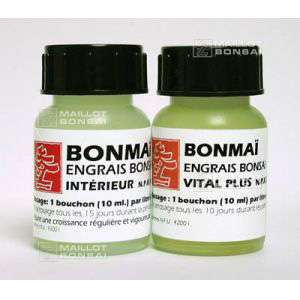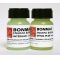##### The Japanese Bonsai specialist
Direct order Contact Help / Services Newsletter# Two pack: 1 vital plus, 1 indoor bonsai food

› Bonsai soil and fertiliser › Fertilizerref. : 5243

6,00

Available quantity : 9Order

###### Description

Bottle: 60ml. N.P.K: 22.18.20. Vital Plus is our bestselling multi-purpose fertiliser to revive all varieties of bonsai trees. Dosage:10 ml per 1 litre of fresh water. Apply every 10 days, especially during periods when your tree may be stressed such as when it's been repotted, lost roots or has dried out.

60 ml bottle. N.P.K 10-10-10. Indoor bonsai will benefit from this liquid chemical fertiliser, which ensures strong, healthy growth and abundant flowers. Bonsai trees are planted in small pots with limited soil and therefore it's important to feed them regularly with nutrients. Dosage: 10 ml or 1 capful of fertiliser per 1 litre of fresh water. Use every 2 weeks.

#bonsai 4.6 #fertiliser 4 #indoor 3.6 #vital 3.5 #plus 3.4 #fertilizer 3 #bottle 2.6 #dosage 2.6 #water 2.5 #litre 2.5

Formule
(( ROUND((CHAR_LENGTH(b.article_nom)-CHAR_LENGTH(REPLACE(b.article_nom, 'bonsai', '')))/LENGTH('bonsai')) + ROUND((CHAR_LENGTH(b.article_description)-CHAR_LENGTH(REPLACE(b.article_description, 'bonsai', '')))/LENGTH('bonsai')) ) * 4.6) + (( ROUND((CHAR_LENGTH(b.article_nom)-CHAR_LENGTH(REPLACE(b.article_nom, 'fertiliser', '')))/LENGTH('fertiliser')) + ROUND((CHAR_LENGTH(b.article_description)-CHAR_LENGTH(REPLACE(b.article_description, 'fertiliser', '')))/LENGTH('fertiliser')) ) * 4) + (( ROUND((CHAR_LENGTH(b.article_nom)-CHAR_LENGTH(REPLACE(b.article_nom, 'indoor', '')))/LENGTH('indoor')) + ROUND((CHAR_LENGTH(b.article_description)-CHAR_LENGTH(REPLACE(b.article_description, 'indoor', '')))/LENGTH('indoor')) ) * 3.6) + (( ROUND((CHAR_LENGTH(b.article_nom)-CHAR_LENGTH(REPLACE(b.article_nom, 'vital', '')))/LENGTH('vital')) + ROUND((CHAR_LENGTH(b.article_description)-CHAR_LENGTH(REPLACE(b.article_description, 'vital', '')))/LENGTH('vital')) ) * 3.5) + (( ROUND((CHAR_LENGTH(b.article_nom)-CHAR_LENGTH(REPLACE(b.article_nom, 'plus', '')))/LENGTH('plus')) + ROUND((CHAR_LENGTH(b.article_description)-CHAR_LENGTH(REPLACE(b.article_description, 'plus', '')))/LENGTH('plus')) ) * 3.4) + (( ROUND((CHAR_LENGTH(b.article_nom)-CHAR_LENGTH(REPLACE(b.article_nom, 'dosage', '')))/LENGTH('dosage')) + ROUND((CHAR_LENGTH(b.article_description)-CHAR_LENGTH(REPLACE(b.article_description, 'dosage', '')))/LENGTH('dosage')) ) * 2.6) + (( ROUND((CHAR_LENGTH(b.article_nom)-CHAR_LENGTH(REPLACE(b.article_nom, 'bottle', '')))/LENGTH('bottle')) + ROUND((CHAR_LENGTH(b.article_description)-CHAR_LENGTH(REPLACE(b.article_description, 'bottle', '')))/LENGTH('bottle')) ) * 2.6) + (( ROUND((CHAR_LENGTH(b.article_nom)-CHAR_LENGTH(REPLACE(b.article_nom, 'water', '')))/LENGTH('water')) + ROUND((CHAR_LENGTH(b.article_description)-CHAR_LENGTH(REPLACE(b.article_description, 'water', '')))/LENGTH('water')) ) * 2.5) + (( ROUND((CHAR_LENGTH(b.article_nom)-CHAR_LENGTH(REPLACE(b.article_nom, 'litre', '')))/LENGTH('litre')) + ROUND((CHAR_LENGTH(b.article_description)-CHAR_LENGTH(REPLACE(b.article_description, 'litre', '')))/LENGTH('litre')) ) * 2.5) + (( ROUND((CHAR_LENGTH(b.article_nom)-CHAR_LENGTH(REPLACE(b.article_nom, 'trees', '')))/LENGTH('trees')) + ROUND((CHAR_LENGTH(b.article_description)-CHAR_LENGTH(REPLACE(b.article_description, 'trees', '')))/LENGTH('trees')) ) * 2.5)

## Secure payment## Delivery

Our logistic partners :04 74 55 23 48
Pépinière MAILLOT-BONSAÏ
Le Bois Frazy
01990 RELEVANT - FRANCE
on appointment